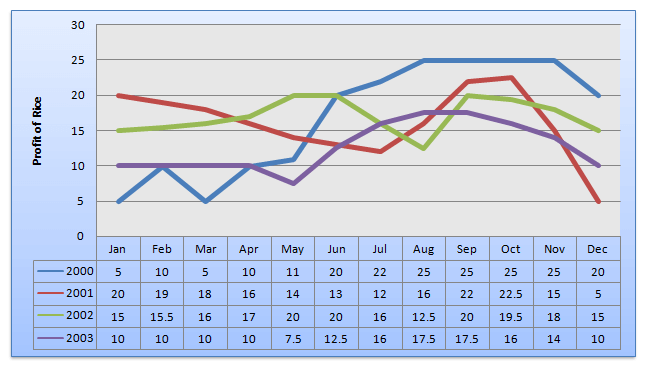Directions: A graph showing the price of rice in India during the years 2000 to 2003 is given below. Study the graph carefully and answer the questions.Question 1: For the month of May, the graph shows that the price of rice was the lowest in the year
(a) 2000
(b) 2001
(c) 2002
(d) 2003

Question 2: The range of price for a year is the difference between the maximum and the minimum prices for that year. The graph shows that this range of price was the greatest for the year
(a) 2000
(b) 2001
(c) 2002
(d) 2003

Question 3: The month in which the prices of rice were equal for the years 2000 and 2002 was
(a) September
(b) August
(c) June
(d) November

Question4: The maximum difference in price between any two years was in the month of
(a) February
(b) November
(c) March
(d) December

### Answers and Explanations

Answer 1: (d) From the graph we see that the price of rice is lowest in 2003 in the month of May.

Answer 2: (a) The lowest price in 2000 is Rs 5 and the highest is Rs 25, so the difference is Rs 20. The lowest price in 2001 is Rs 5 and the highest is Rs 23, so the difference is Rs 18. The lowest price in 2002 is Rs 13 and the highest is Rs 20, so the difference is Rs 7. The lowest price in 2003 is Rs 7 and the highest price is Rs 17, so the difference is Rs 10. Hence the difference is maximum in the year 2000.

Answer 3: (c) The month in which price of rice was equal in 2000 and 2002 is June.

Answer 4: (d) It can be observed that among the different options, December is the required month.